$$\def\RR{\bf R} \def\real{\mathbb{R}} \def\bold#1{\bf #1} \def\d{\mbox{Cord}} \def\hd{\widehat \mbox{Cord}} \DeclareMathOperator{\cov}{cov} \DeclareMathOperator{\var}{var} \DeclareMathOperator{\cor}{cor} \newcommand{\ac}{\left\{#1\right\}}$$

## Estimating Causal Pathway Effects via Large-scale Multilevel Models

### Xi (Rossi) Luo

Brown University
Department of Biostatistics
Center for Statistical Sciences
Computation in Brain and Mind
Brown Institute for Brain ScienceCCNS Workshop at SAMSI
May 4, 2016

Funding: NSF/DMS (BD2K) 1557467; NIH P20GM103645, P01AA019072, P30AI042853; AHA

## CoauthorYi Zhao
(3rd Yr PhD Student)
Brown University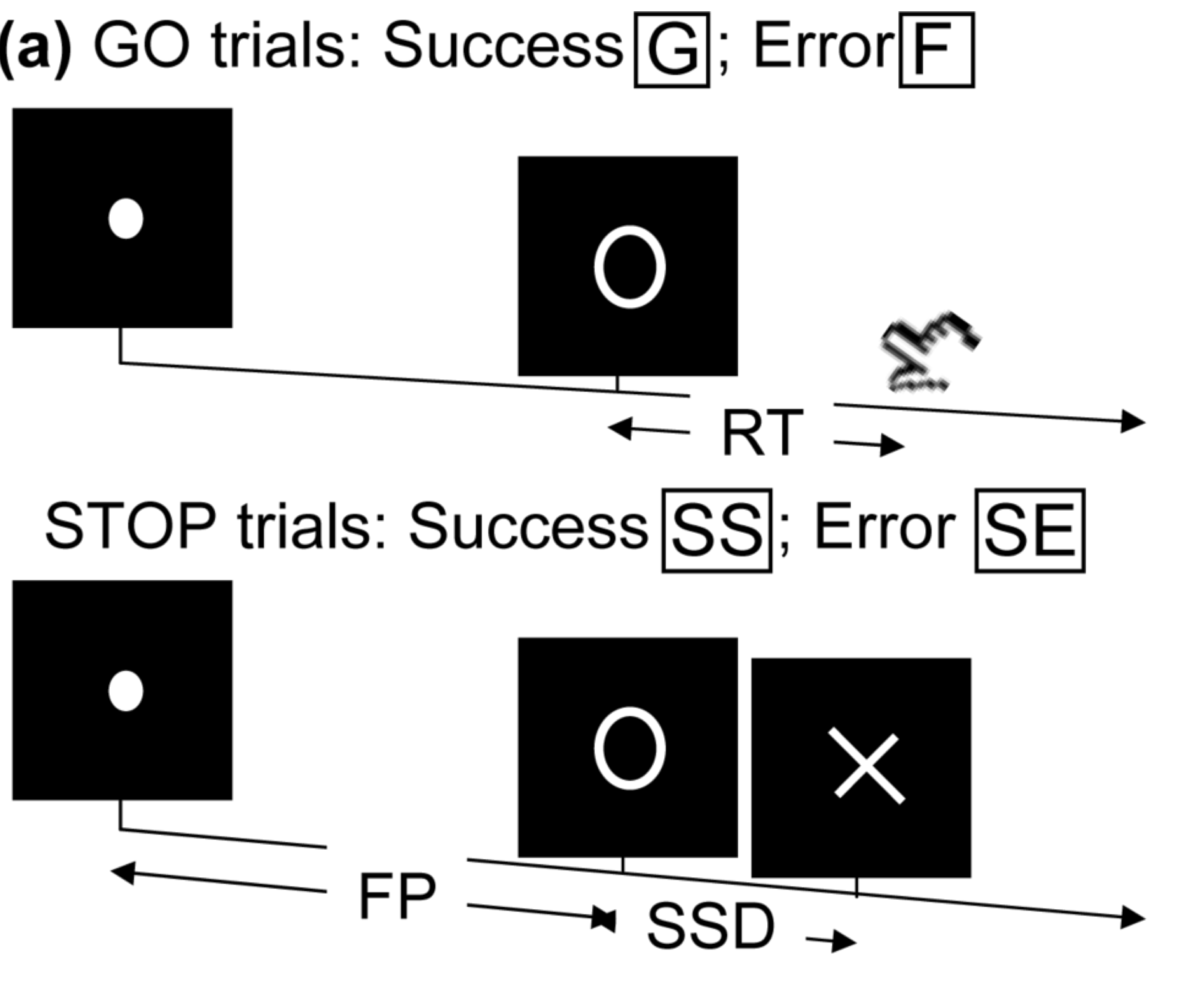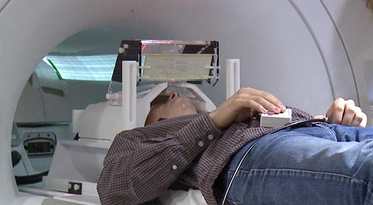• press button if "go";
• withhold pressing if "stop"
• Not resting-state: "rest" in scanner

Goal: causal mechanisms from stimuli to motor cortex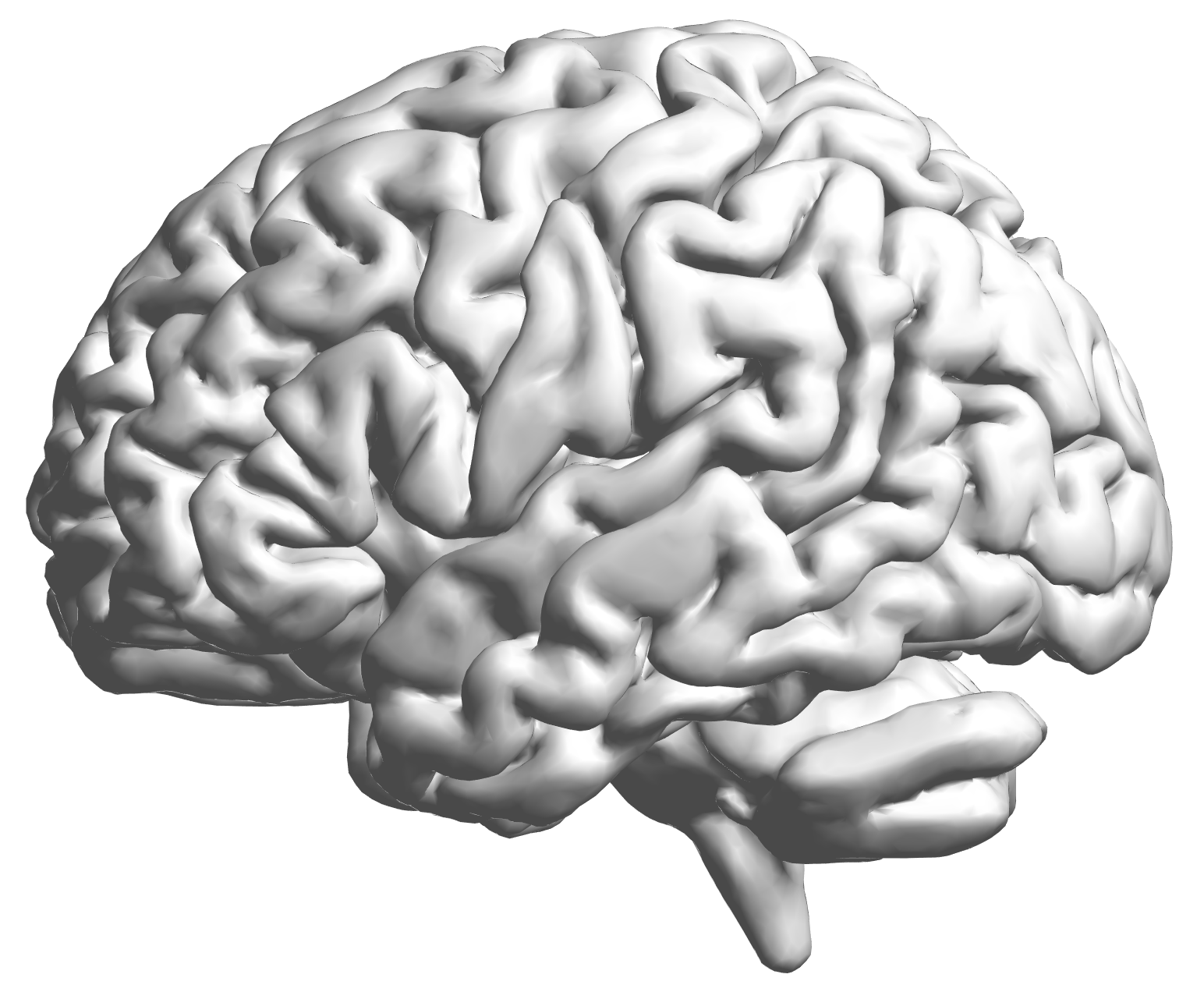fMRI data: blood-oxygen-level dependent (BOLD) signals from each cube/voxel (~millimeters), $10^5$ ~ $10^6$ voxels in total.

## Raw Data: Motor Region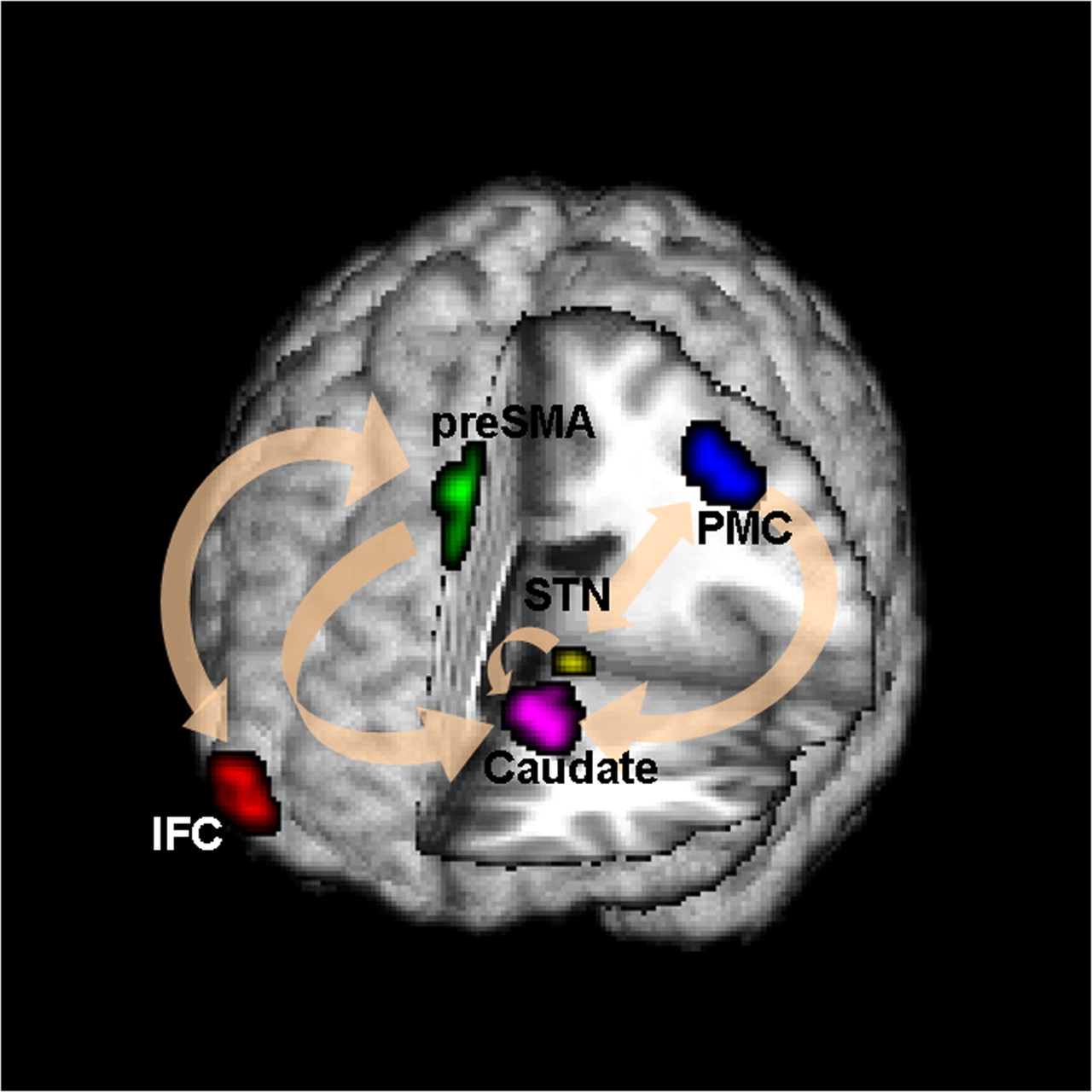Time (seconds)

Black: fMRI BOLD activity
Blue: stop onset times; Red: go onset times

## fMRI Studies

Sub 1, Sess 1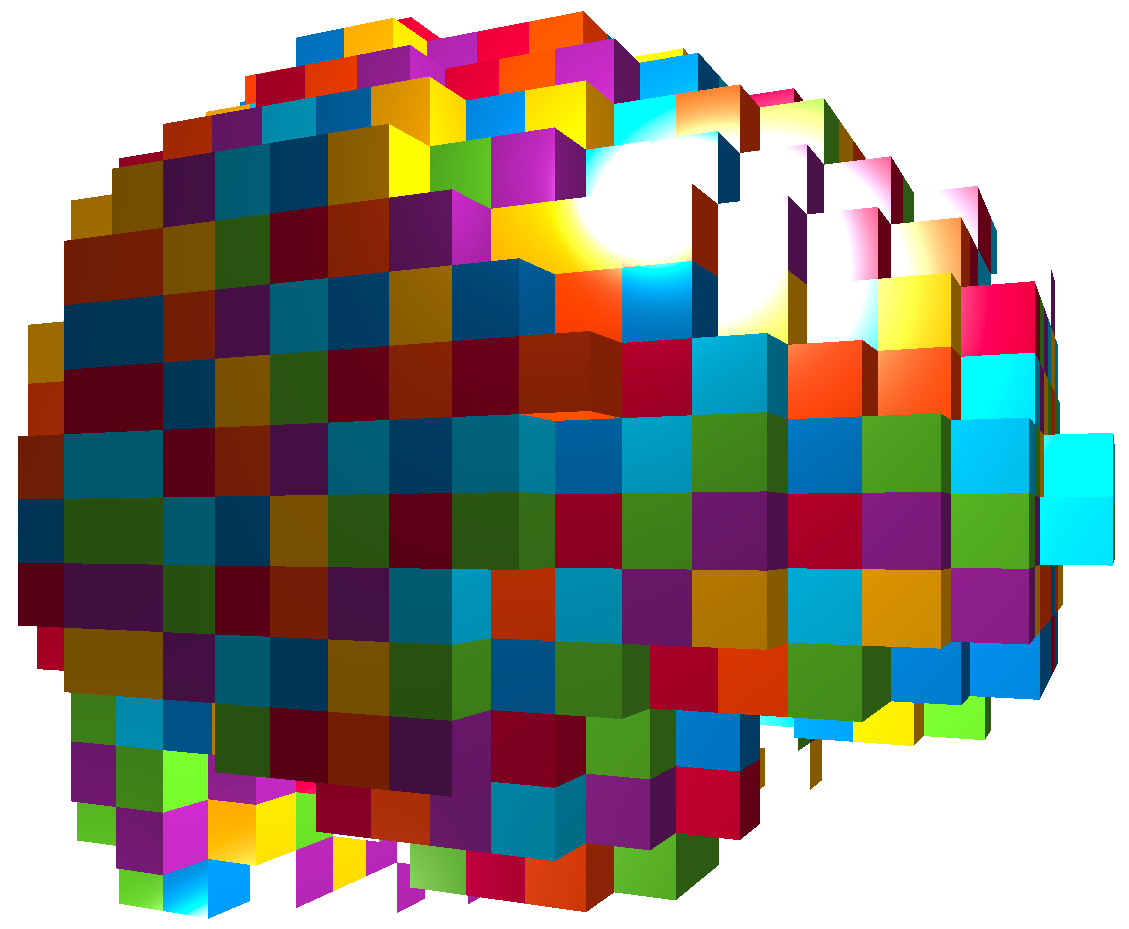Time 12~300

Sub i, Sess jSub ~100, Sess ~4Our data: 98 subjects × 4 sessions × 100 trials × 2 regions

Question: can "big and complex" fMRI data be helpful?

## Brain Networks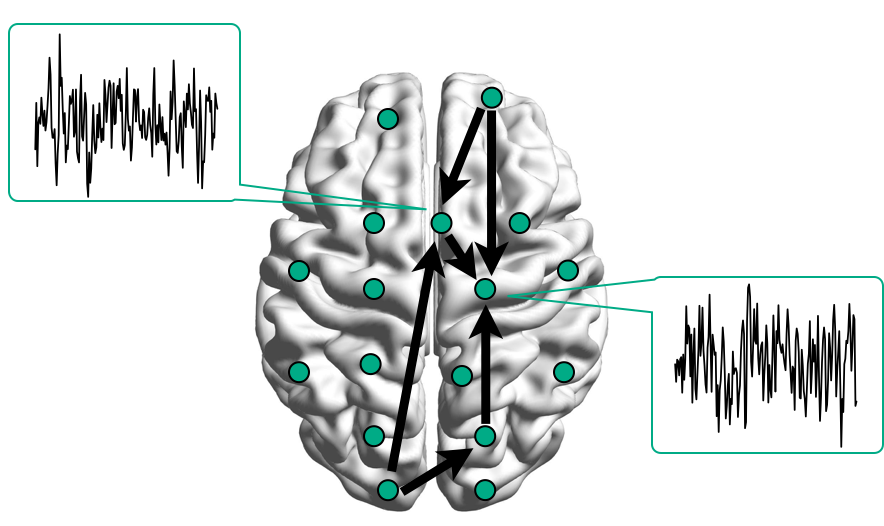Functional/Effective Connectivity

nodes/connections removed to enhance visualization

## Network Model with Stimulus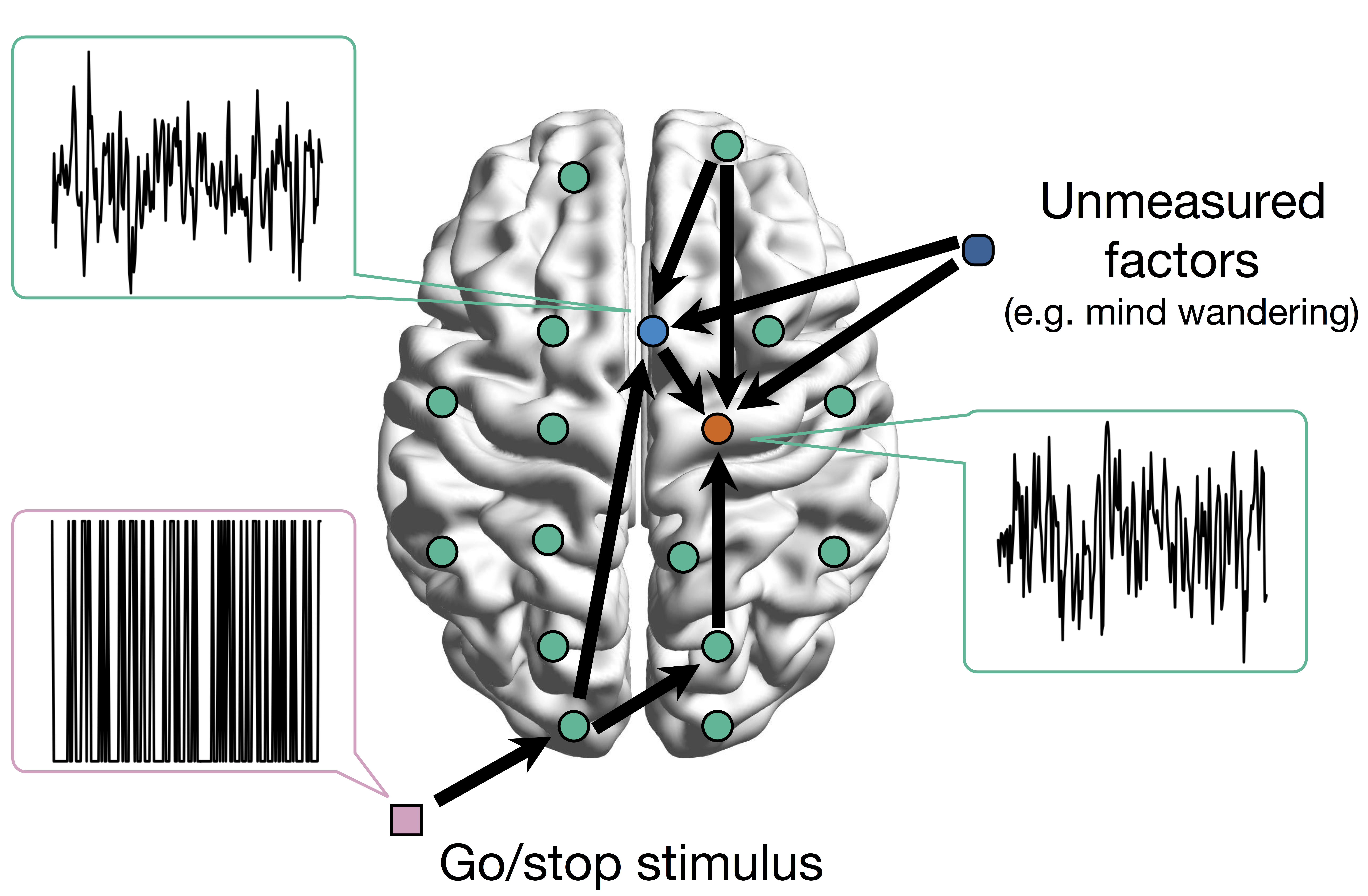Goal: quantify effects stimulipreSMAPMC regions Duann, Ide, Luo, Li (2009). J of Neurosci

# Model

## Mediation Analysis and SEM\begin{align*}M &= Z a + \overbrace{U + \epsilon_1}^{E_1}\\ R &= Z c + M b + \underbrace{U g + \epsilon_2}_{E_2}, \quad \epsilon_1 \bot \epsilon_2\end{align*}
• Indirect effect: $a \times b$; Direct effect: $c$
• Correlated errors: $\delta = \cor(E_1, E_2) \ne 0$ if $U\ne 0$

## Mediation Analysis in fMRI

• Mediation analysis (usually assuming $U=0$)
• Baron&Kenny, 86; Sobel, 82; Holland 88; Preacher&Hayes 08; Imai et al, 10; VanderWeele, 15;...
• Parametric Wager et al, 09 and functional Lindquist, 12 mediation, under (approx.) independent errors
• Stimulus $\rightarrow$ brain $\rightarrow$ user reported ratings, one brain mediator
• Assuming $U=0$ between ratings and brain
• Multiple mediators and multiple pathways
• Dimension reduction by arXiv1511.09354Chen, Crainiceanu, Ogburn, Caffo, Wager, Lindquist, 15
• Pathway Lasso penalization Zhao, Luo, 16
• This talk: causal estimation under $U\ne 0$ (its effect size $\delta \ne 0$) when modeling two brain regions## Existing Approaches for $\delta \ne 0$

• Assuming $\delta=0$
• Assumption "too strong" for most cases Imai et al, 10
• Sensitivity plot: "guessing" $\delta$
• Simplify models: e.g. $c=0$ via instrumental variable
• Adjust if possible Sobel, Lindquist, 14
• Use Bayesian prior or regularizationEffects can be positive or negative depending on $\delta$

# Method

## Our Approach: Step 1

• Bivariate single-level model \begin{align*}\begin{pmatrix}M & R\end{pmatrix} & = \begin{pmatrix}Z & M\end{pmatrix} \begin{pmatrix}a & c\\ 0 & b \end{pmatrix} + \begin{pmatrix}E_{1} & E_{2}\end{pmatrix}\\ (E_1, E_2) & \sim MVN(\boldsymbol{0}, \boldsymbol{\Sigma}) \quad \boldsymbol{\Sigma}=\begin{pmatrix}\sigma_{1}^{2} & \delta\sigma_{1}\sigma_{2}\\ \delta\sigma_{1}\sigma_{2} & \sigma_{2}^{2} \end{pmatrix} \end{align*}
• Estimate $(a,b,c,\boldsymbol{\Sigma})$ via ML (a lot of handwaving)
• We solve an optimization with constraints
• Different than running two regressions

## Causal Interpretation

• Prove causal using potential outcomes Neyman, 23; Rubin, 74
• Causal inference intuition $$Z \rightarrow \begin{pmatrix} M \\ R \end{pmatrix}$$
• Other approaches assume $$r_{i}\left(z_{i}^{\prime},m_{i}\right)\bot m_{i}\left(z_{i}\right)|Z_{i}=z_{i}$$
• We do not need this assumption

## Theory

• Theorem: Given $\delta$, unique maximizer of likelihood, expressed in closed form
• Theorem: Given $\delta$, our estimator is root-n consistent and efficient
• Bias (and variance) depends on $\delta$

[Stigler 2007]

# "Tragedy" of ML

Theorem: The maximum likelihood value is the same for every $\delta \in (-1,+1)$.• Likelihood provides zero info about $\delta$
• Cannot simply use prior on $\delta$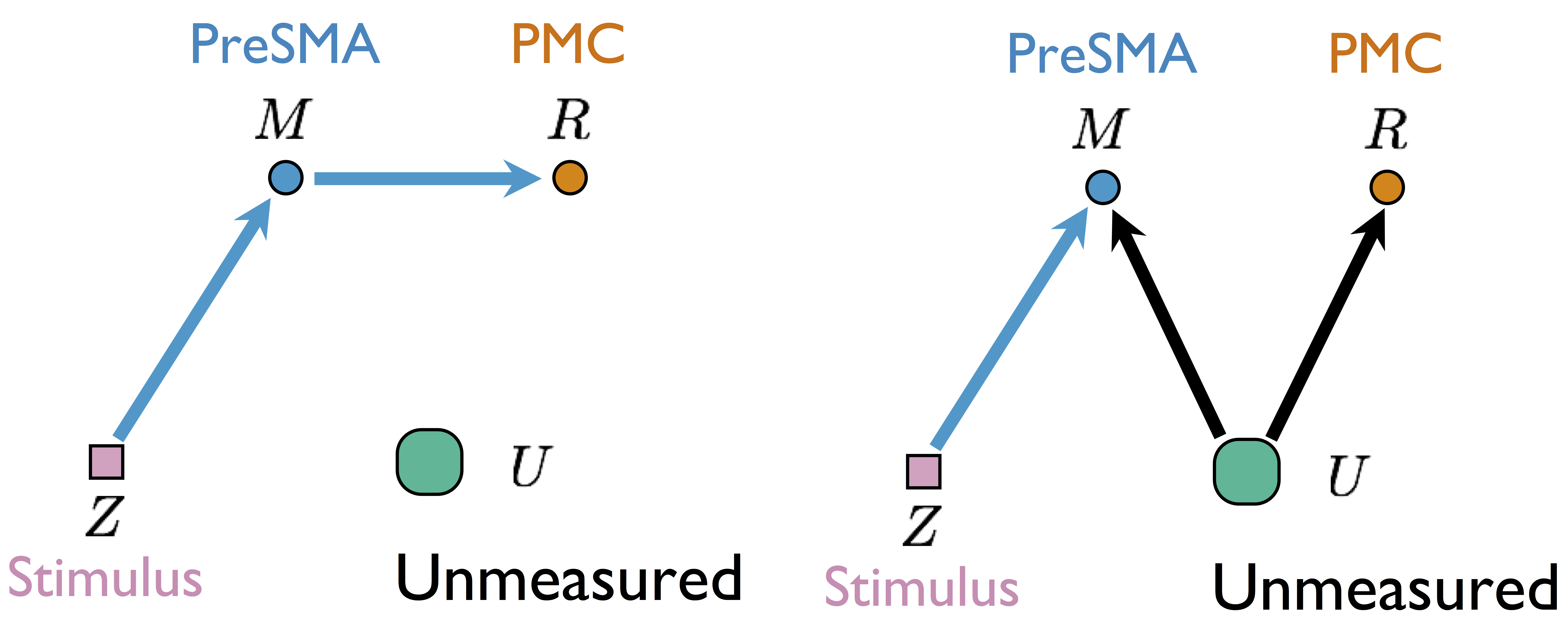Two different models generate same single-trial BOLD activations if only observing $Z$, $M$, and $R$

without measuring $U$

## Our Approach: Step 2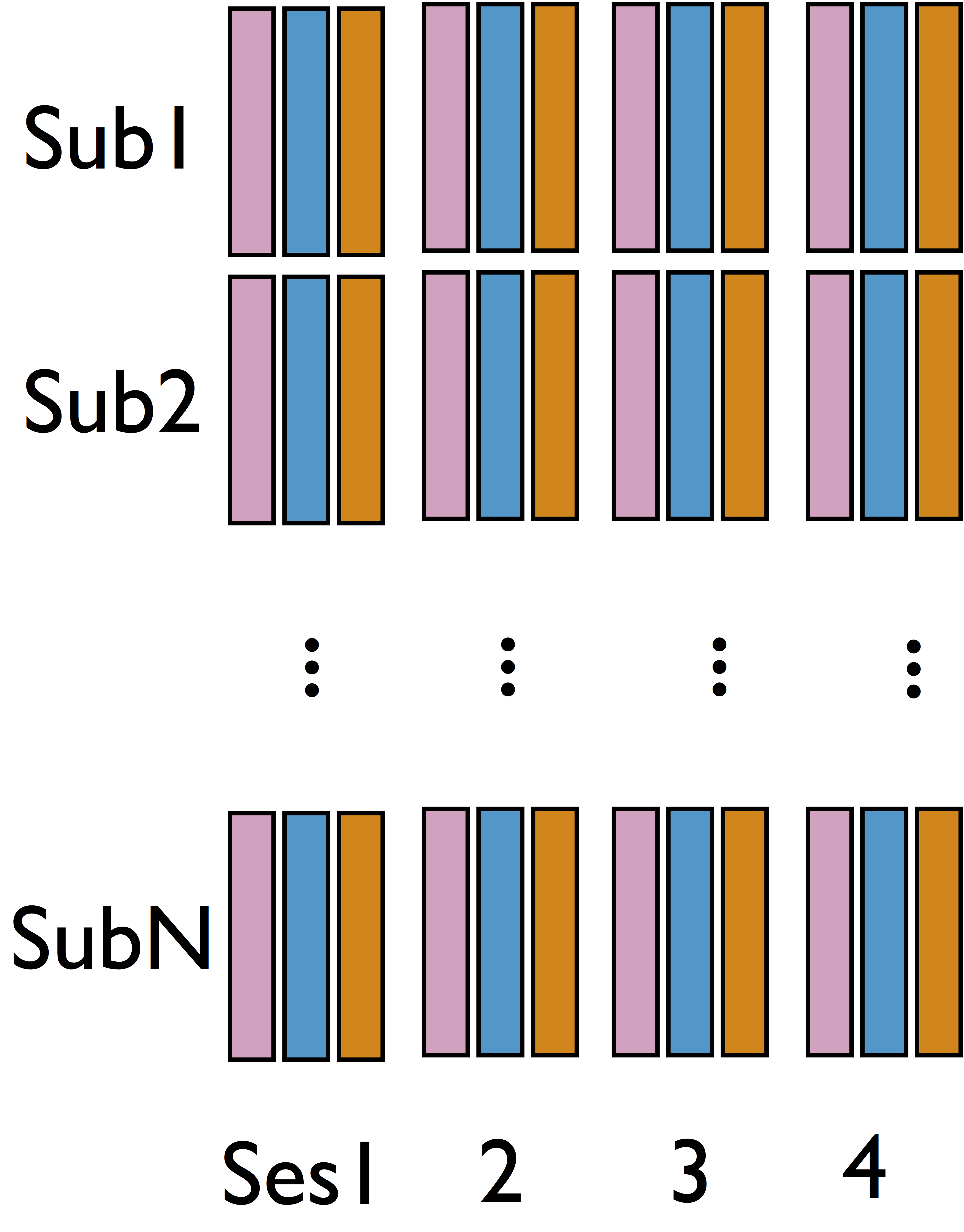• Cannot identify $\delta$ from single sub and single sess (see our theorem)
• Intuition: leverage complex data structure to infer $\delta$

## Step 2: Some Details

• Step 1 model for each sub and each sess $$\begin{pmatrix}{M}_{ik} & {R}_{ik}\end{pmatrix}=\begin{pmatrix}{Z}_{ik} & {M}_{ik}\end{pmatrix}\begin{pmatrix}{a}_{ik} & {b}_{ik}\\ 0 & {c}_{ik} \end{pmatrix}+\begin{pmatrix}{E}_{1_{ik}} & {E}_{2_{ik}}\end{pmatrix}$$
• Limited variability in $\delta$ across sub/sess
• Random effect model cf AFNI, FSL, SPM, and etc $$\begin{pmatrix}{A}_{ik}\\ {B}_{ik}\\ {C}_{ik} \end{pmatrix}=\begin{pmatrix}{A}\\ {B}\\ {C} \end{pmatrix}+\begin{pmatrix}\alpha_{i}\\ \beta_{i}\\ \gamma_{i} \end{pmatrix}+\begin{pmatrix}\epsilon_{ik}^{{A}}\\ \epsilon_{ik}^{{B}}\\ \epsilon_{ik}^{{C}} \end{pmatrix}=b+u_{i}+\eta_{ik}$$

## Option 1: Two-stage Fitting

• Stage 1: fit $(\hat{A}_{ik}(\delta), \hat{B}_{ik}(\delta), \hat{C}_{ik}(\delta))$ for each $i$ and $k$ for varying $\delta$ using our step 1 single-level model
• Stage 2: Find $\hat{\delta}$ that $(\hat{A}_{ik}(\hat{\delta}), \hat{B}_{ik}(\hat{\delta}), \hat{C}_{ik}(\hat{\delta}))$ yields maximum likelihood for random effects model
• Small-scale computing
• However, estimation error in stage 1 not accounted
• Complicates our goal on determining the variance

## Option 2: Integrated Modeling

• Optimize all parameters in joint likelihood \begin{align*} &\sum_{i=1}^{N}\sum_{k=1}^{K}\log\Pr\left(R_{ik},M_{ik}|Z_{ik},\delta,b_{ik},\sigma_{1_{ik}},\sigma_{2_{ik}}\right)\quad \mbox{Data}\\ & + \sum_{i=1}^{N}\sum_{k=1}^{K}\log\Pr\left(b_{ik}|u_{i},b,\boldsymbol{\Lambda}\right)\quad \mbox{Subject variation}\\ & +\sum_{i=1}^{N}\log\Pr\left(u_{i}|\boldsymbol{{\Psi}}\right) \quad \mbox{Prior}\end{align*}
• Large computation: $5NK + 3N + 11 > 2000$ paras

## Algorithm

Theorem: The joint likelihood is conditional convex for groups of parameters.
Algorithm: block coordinate descent with projections.

Leverage conditional convexity to reduce computation

details in our paper

# Simulations

## Method Comparison

• Single level models
• BKBaron, Kenny, 86: assuming $\delta = 0$
• CMA-$\delta$ (our single-level method): assuming $\delta = 0$
• Mixed effects multilevel models
• KKBKenny et al, 03: assuming all $\delta = 0$
• CMA-ts (ours): estimating $\delta$, two-stage fitting
• CMA-h, CMA-m (ours): estimating $\delta$, large-scale fitting
• Simulate data with varying $\delta$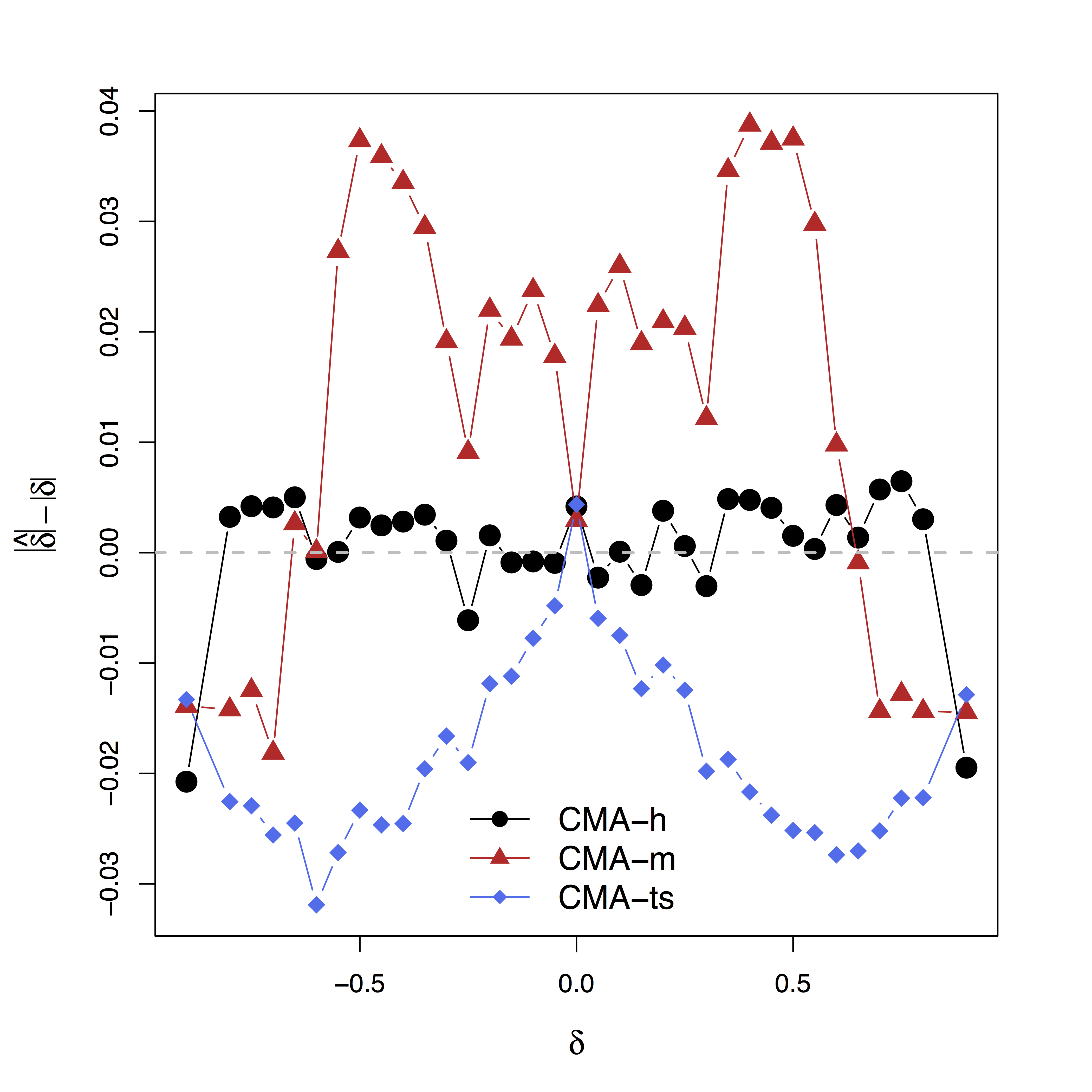Low bias for $\delta$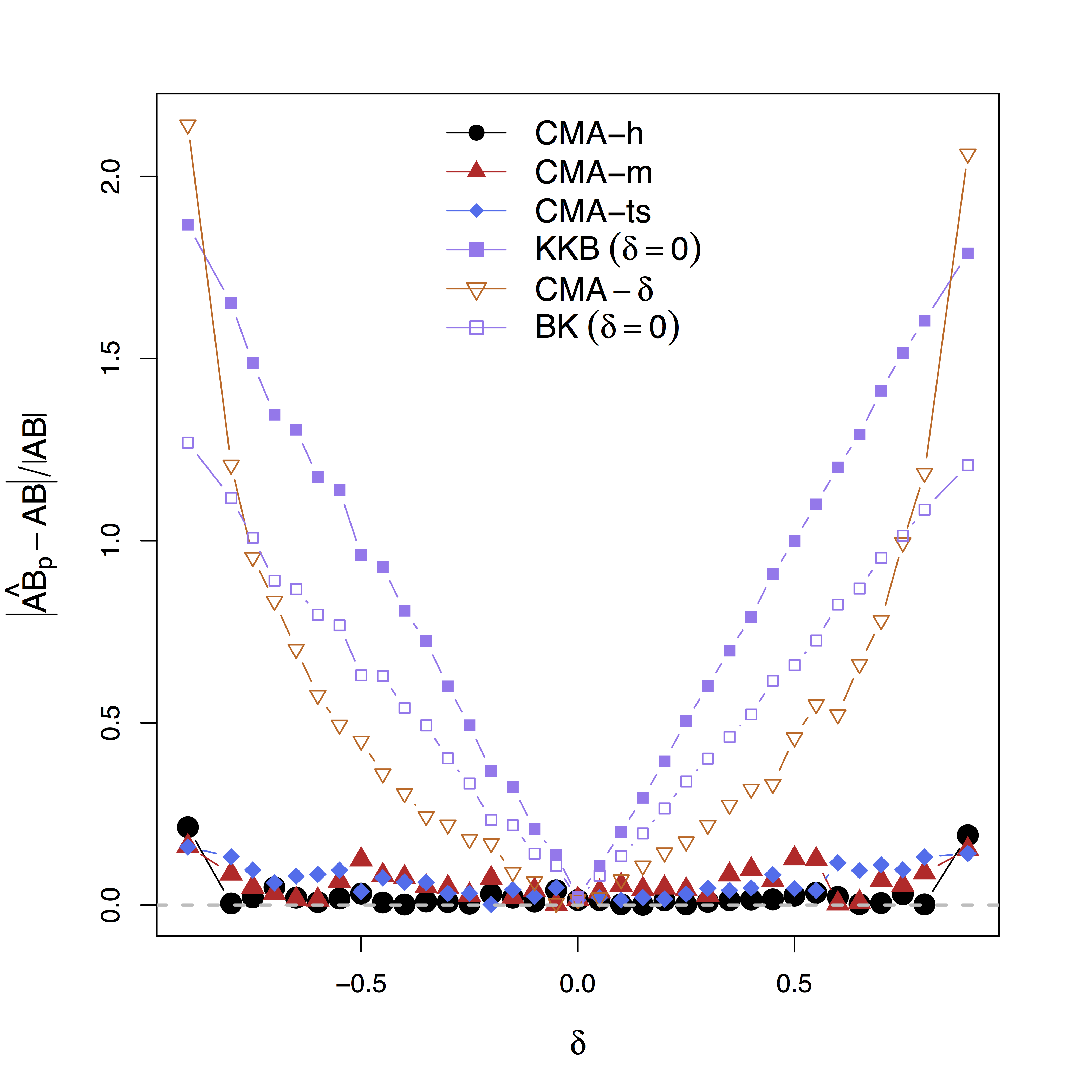Low bias for effects

Our large-scale multilevel model performs best; Bias can be large (100%) without estimating $\delta$

# Real Data

## Data

• Random stimuli and scalar activations for each trial
• Stimuli: go = press; stop = not pressing
• stop is expected to suppress motor area (PMC)
• 98 subjects × 4 sessions × 100 trials × 2 regions
• Temporal dependence removed using whitened data and single-trial beta deconvolutionWager et al, 08

## Identifiability and Bias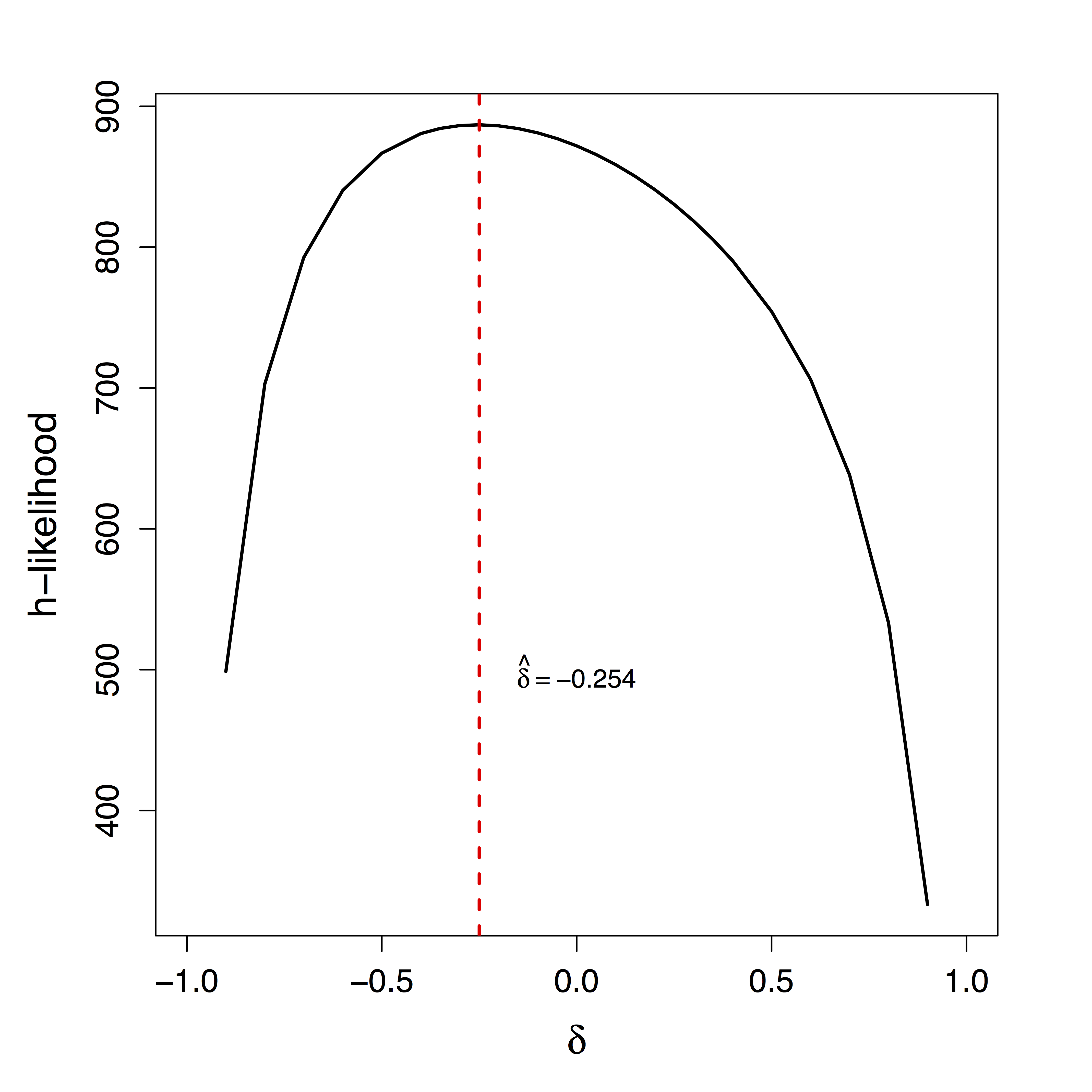Unique $\delta$ for ML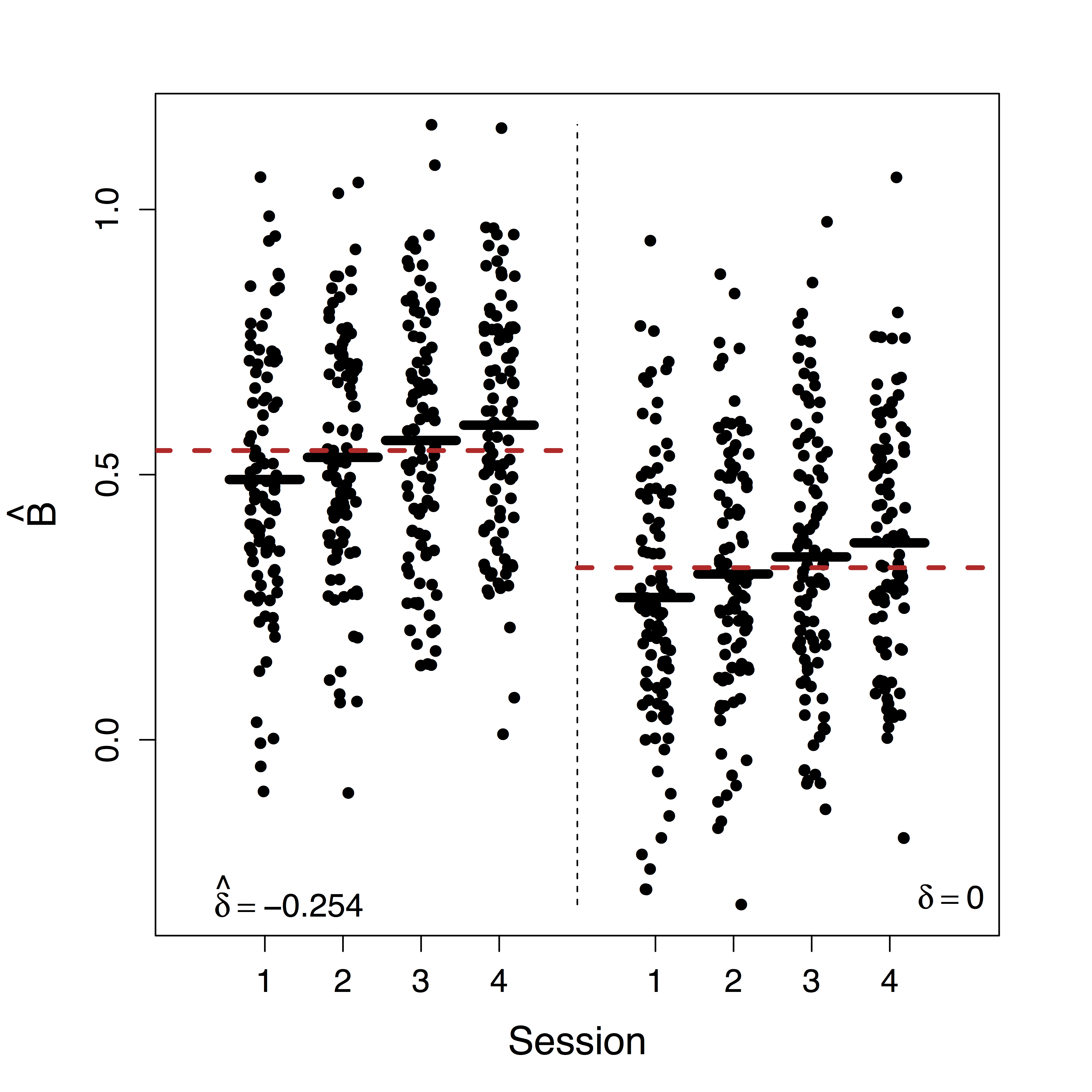Estimates doubled• Ours show stop directly suppresses PMC significantly while others do not recover this
• Motion (part of $U$) correction decreases our $\delta$ estimate but do not change our causal estimates

## Summary

• Leverage complex data for causal fMRI modeling
• Approach: large-scale causal SEM + big multilevel data + machine learning/optimization
• Theory: identifiability, convergence, and computation
• Result: reduced bias and improved interpretation
• Extensions: temporal models, identifiability conditions, multiple pathwaysPathway Lasso, our arXiv 1603.07749
• Manuscript: Consistent Mediation Analysis, 2015 ENAR Student Paper Award, revision for JASA, (arXiv 1410.7217)

# Thank you!

## Slides at: bit.ly/xlSAMSI16